## An astronaut holds a rock 100 m above surface of Planet X. The rock is then thrown upwards with a sleek of 15m/s. The rock reaches the groun

Question

An astronaut holds a rock 100 m above surface of Planet X. The rock is then thrown upwards with a sleek of 15m/s. The rock reaches the ground 10s after it is thrown the atmosphere of Planet X has a negligible effect on the rock. Determined the acceleration due to gravity of the rock when it is in planet x

in progress 0
6 months 2021-08-09T16:46:34+00:00 1 Answers 5 views 0

1. Answer: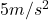Explanation:

This problem is related to vertical motion, and the equation that models it is: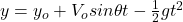(1)

Where: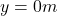is the rock’s final height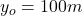is the rock’s initial height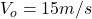is the rock’s initial velocity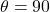is the angle at which the rock was thrown (directly upwards)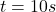is the time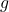is the acceleration due gravity in Planet X

Isolatingand taking into account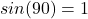: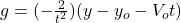(2)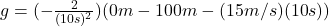(3)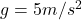(4) This is the acceleration due gravity in Planet X